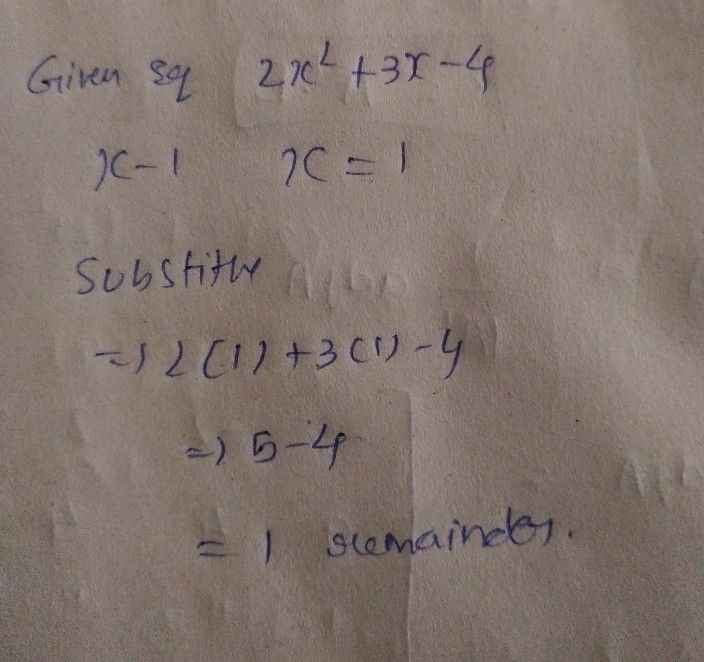Symbol
ProblemWhat is the remainder when $2x^{2}+3x-4$ 4 $1s$ divided by $y$ $\left(x-1\right)3$